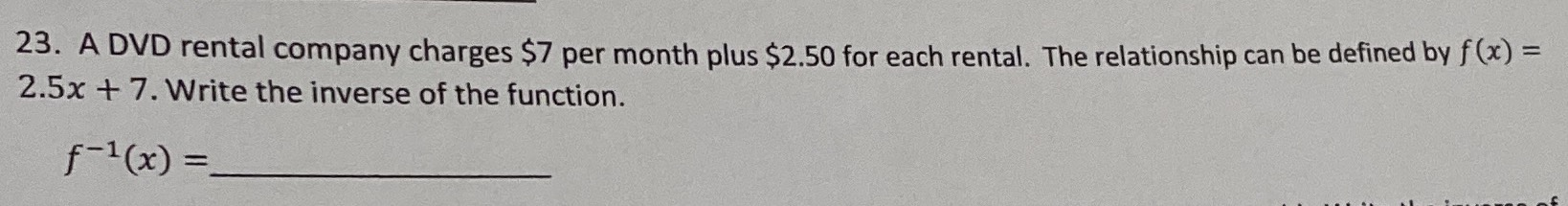### ¿Todavía tienes preguntas de matemáticas?

Pregunte a nuestros tutores expertos
Algebra
PreguntaA DVD rental company charges $$\ 7$$ per month plus $$\ 2.50$$ for each rental. The relationship can be defined by $$f ( x ) =$$ $$2.5 x + 7$$ . Write the inverse of the function. $$f ^ { - 1 } ( x ) =$$

$$\frac{2}{5} x- \frac{14}{5}$$